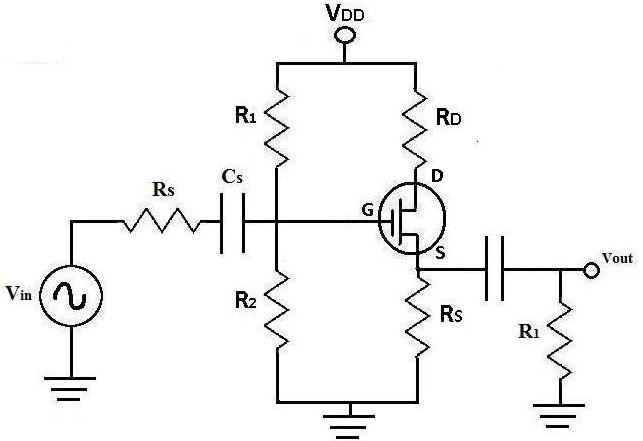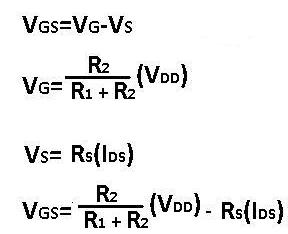﻿ DC Analysis of a MOSFET Transistor Circuit# DC Analysis of a MOSFET Transistor CircuitShown above is a typical MOSFET transistor circuit.

We're going to now show how to perform DC analysis on this MOSFET circuit so that we can find crucial DC values of the circuit.

When doing DC analysis, all AC voltage sources are taken out of the circuit because they're AC sources. DC analysis is concerned only with DC sources. We also take out all capacitors because in DC, capacitors function as open circuits. For this reason, everything before and after capacitors are removed, which in this circuit includes resistor, Rs.

Below is the schematic of the DC equivalent of the mosfet circuit above:Now let's do the calculations for DC analysis. Vgs is the voltage that falls across the gate and the source of the mosfet transistor. It is crucial to calculate because in order to solve for Ids, the current from the drain to the source, Vgs must be known.

In order to solve for Vgs, Vg, the voltage at the gate, and Vs, the voltage at the source must be known:Now that we've solved for Vgs, we now solve for Ids. The formula for Ids is:Kn and Vtn are normally givens that you just plug into the equation.

Once you solve for Ids, you will get two currents from solving that quadratic equation. The current that produces a Vgs which is greater than Vtn is the real current of the circuit and the other should be eliminated.

Now we calculate Vds and the Q-point: